Learn to Write DAX in Power BI - Excelerator BI

# Introduction

DAX in both Excel and Power BI follow the same syntax and semantics. So, whether you write DAX in Excel or in Power BI, the DAX formulas are the same,  the only difference is in the user interface of Excel and Power BI. Excel Pros benefit by learning to write DAX in Excel. However, those who would like to learn to write DAX in Power BI, can use this guide as a reference to practice the examples and exercises given in my book – Learn to Write DAX.

So, if you want to write DAX in Power BI, read the following tips on how to use Power BI Desktop instead of the Excel user interface.

## Setting Up a Pivot Table

In Power BI Desktop, a good substitute to an Excel Pivot Table is the Matrix visual in a Report.

You use four areas of the Fields list to create or update a Matrix: Filters (#1 in the figure below), Columns (#2), Rows (#3), Values (#4).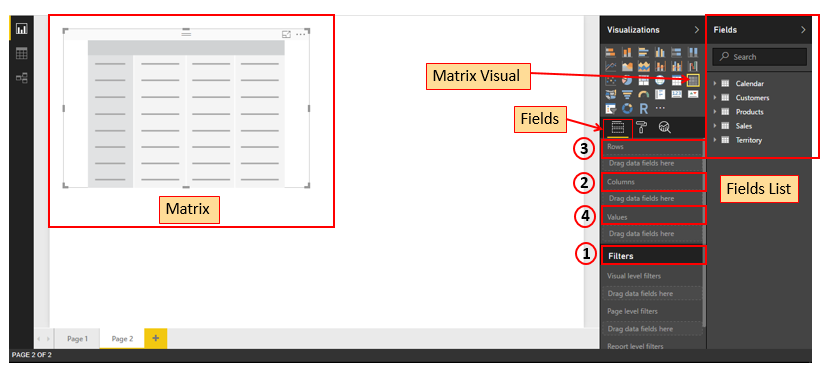Say that the instructions in this book tell you to set up a pivot table with Product[Category] on Rows, Customer[Gender] on columns, Calendar[CalendarYear] on Filters, and a calculated field such as [Total Sales Amount] on Values.

In that case, you should then use the Fields list (shown on the right below) to build the Matrix (shown on the left below) as instructed.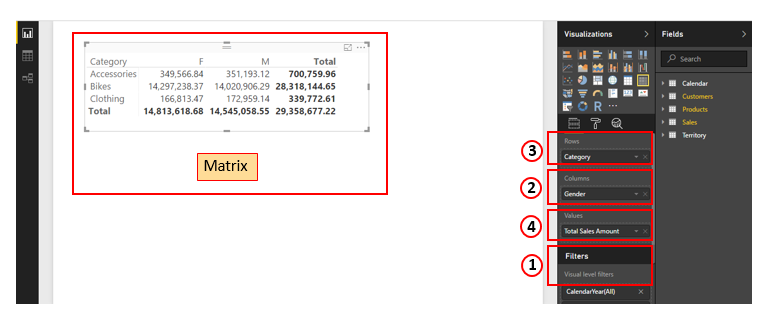In Power BI, a Slicer is also a visual. So, if you want Customer[Occupation] on a Slicer, place the Slicer visual next to the above Matrix visual and add Customer[Occupation] to it.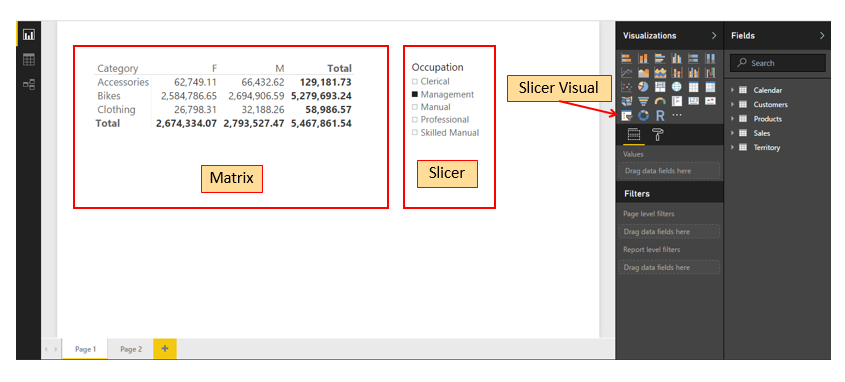## Naming Conventions

The best practices in the naming conventions remain the same in both Excel and Power BI.

### Syntax for Writing DAX Formulas in Power BI

In Power BI, whether you click on New Measure on the Ribbon under Modelling tab or right-click on the table name in the fields list and then select the New measure, you can create the measure in the formula bar as follows, and the same syntax is used throughout the book.

Total Sales = SUM(Sales[ExtendedAmount])

# 1: Concept: Pivot Tables vs. Power Pivot vs. Power BI

In Excel, the data model, comprising of the tables and the relationships between the tables can be viewed in the Power Pivot window. The metadata in the form of measures and/or calculated columns can be created using DAX formulas in the Excel window, as I do in my book, but ultimately the metadata gets combined into the data model.

In Power BI Desktop, you will not find any mention of either Power Pivot or data model, but beneath the hood of the user interface, the data model is the same as that of in Excel. And, in fact, a data model created in one of Excel workbook or Power BI workbook, can be shared with the other.

You can do the data modelling in Power BI with the same DAX formulas that I explain throughout my book. And, once again, the difference is only the User Interface that you will learn in this guide.

## Power BI Desktop User Interface

In Power BI Desktop, you will find three icons in the left panel – Report, Data, and Relationships.

Report – In the Report view, you can have different visualisations of the data. On the Ribbon, you can find the data modelling commands under the Modelling tab.

In this view, you can have the Matrix visual that resembles the Excel Pivot Table, to follow through the examples in my book.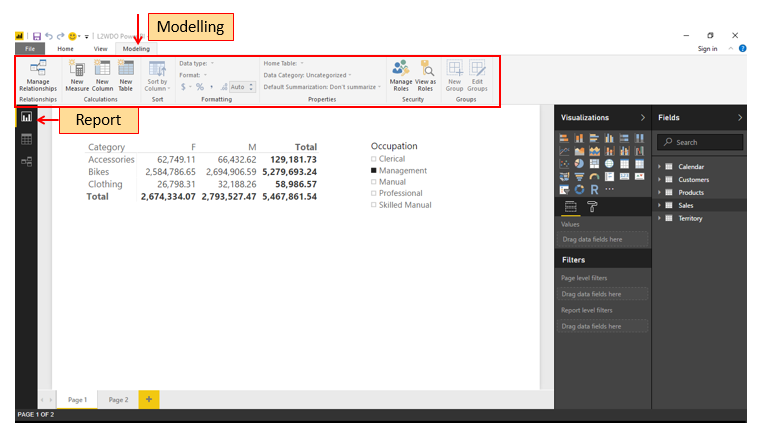Data – In the Data view, you will find all the tables loaded into the data model. On the Ribbon, you can find the data modelling commands under the Modelling tab.

This view is similar to the Data view in the Power Pivot window in Excel.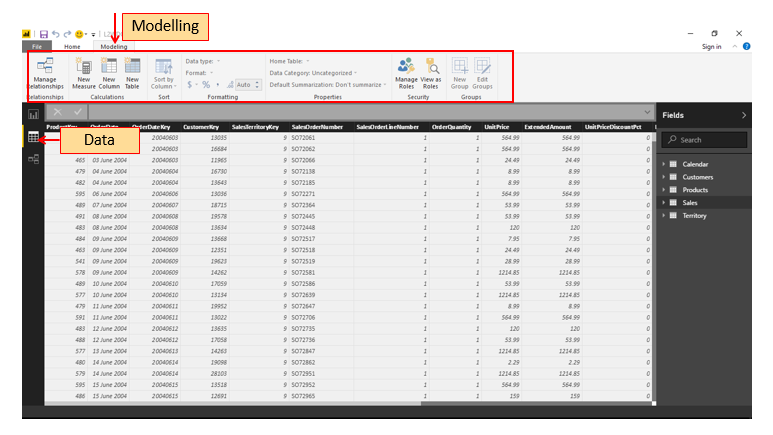Relationships – In the Relationships view, you will find all the tables loaded into the data model and the relationships between them. You can create and manage the relationships in this view. On the Ribbon, you can find the data modelling commands under the Modelling tab.

This view is similar to the Diagram view in the Power Pivot window in Excel.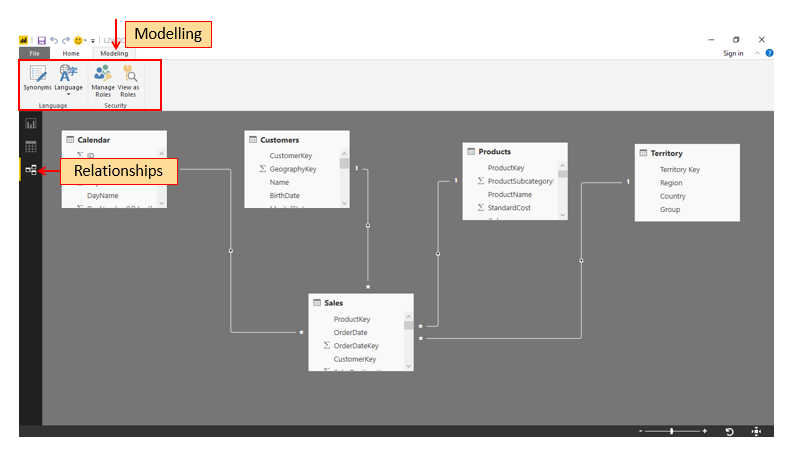# 2: Concept: Loading Data

In Power BI Desktop, you can load the required data directly into the data model.

## Here’s How: Loading Data from a New Source

You can download a copy of the sample AdventureWorks database used in this book from http://xbi.com.au/learndax.

1. You should download the database now, unzip it, and place it in a location that is easy for you to find.

You are going to start off by loading the following tables from the AdventureWorks Access database:

• Sales
• Products
• Territories
• Calendar
• Customers

Follow these steps to load data into a Power BI:

1. Open a new blank Power BI workbook.
2. Select Home, Get Data (see #1 below), More … (#2).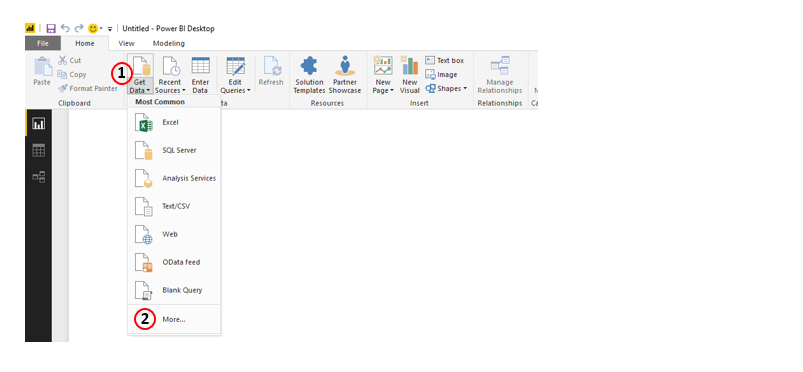4. In the Get Data dialog box (see #3 below), select Database (#4), Access database (#5), and then click on Connect (#6).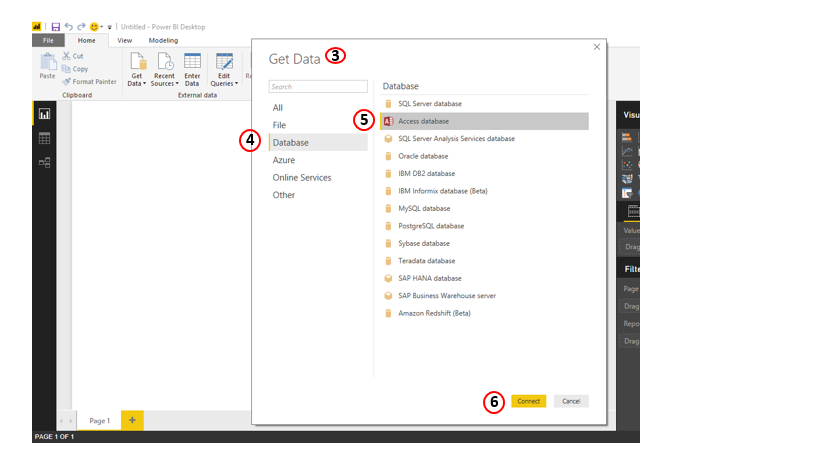5. Browse to the location of the sample database you downloaded and unzipped in step 1 and then click Open.

6. Navigator window appears as shown below (#7). Select the tables by clicking in the check boxes. Then, click on Load (#8).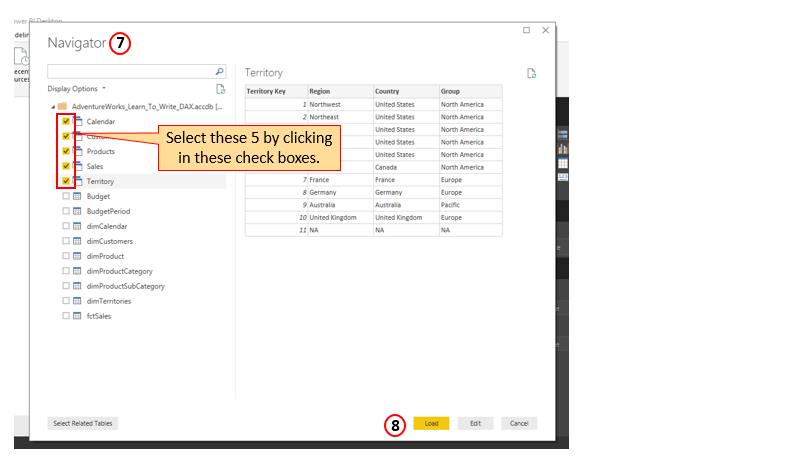The load progress is displayed, and this may take a little while.

7. Right-click on the Territory tab and rename it Territories for consistency.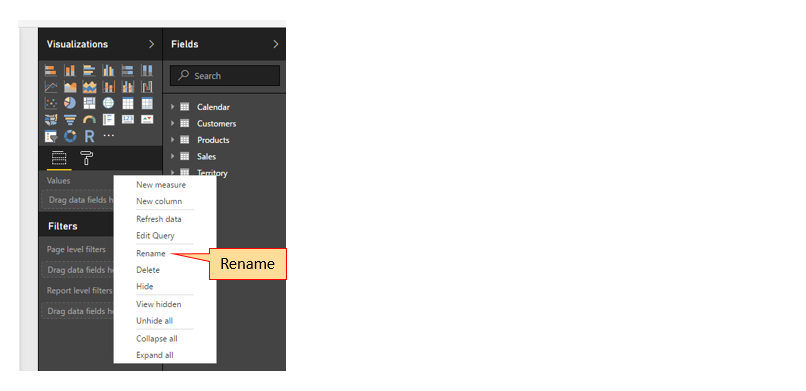## Relationships Between Tables

Click on the Relationships icon in the left panel.

The steps to create relationships are the same as in Power Pivot and you can follow through my instructions in the book.

## Here’s How: Making Changes to a Table That Is Already Loaded

Say that you want to make changes to the Calendar table so that you only bring in dates for the years 2002 and 2003, and you also want to remove the fiscal date columns from the table. The following steps walk you through how to make changes like these to a table that is already loaded:

1. In the home tab, click on Edit Queries (see #1 below) on the Ribbon.Query Editor window appears.

1. In the Queries list (#2), click on Calendar.
2. Click the arrow at the right end of the CalendarYear column (#3).
3. Click on Load more (#4) to view the complete list.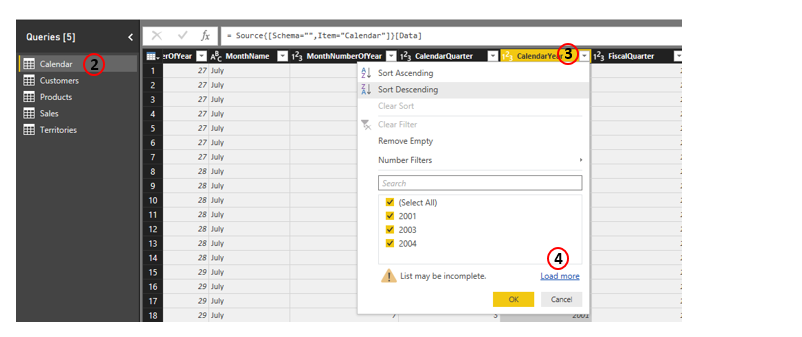5. Deselect years 2001 and 2004 (#5 and #6) and then click OK.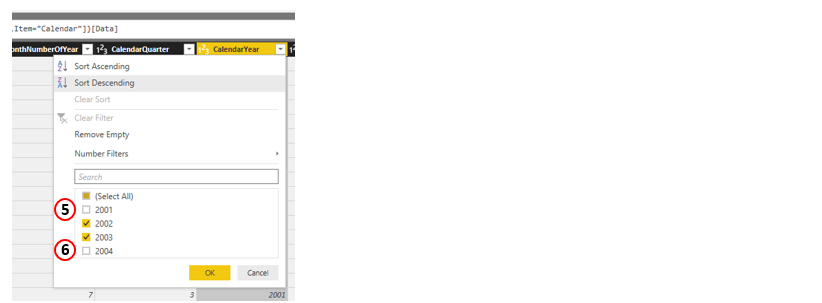6. Click on Choose Columns (#7) on the Ribbon.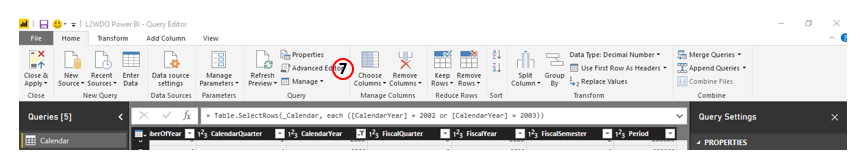7. In the Choose Columns dialog box that appears, deselect the three fiscal columns by clicking on the checkboxes (#8), and then click OK.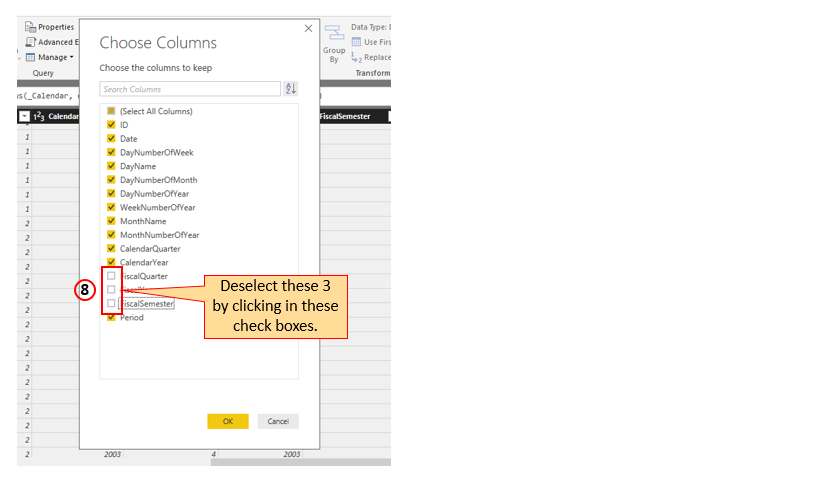9. Click on Close & Apply (#9) on the Ribbon.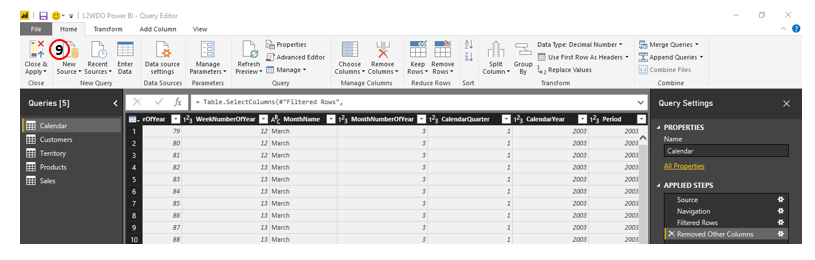Save the workbook.

## Here’s How: Importing New Tables from an Existing Connection

In this exercise, you will open the existing connection to your Access database, bring in the ProductSubCategory table, and join it to your data model. Follow these steps:

1. On the Home tab, click on Existing Sources (see #1 below).

2. Click on the Access connection you created earlier (#2).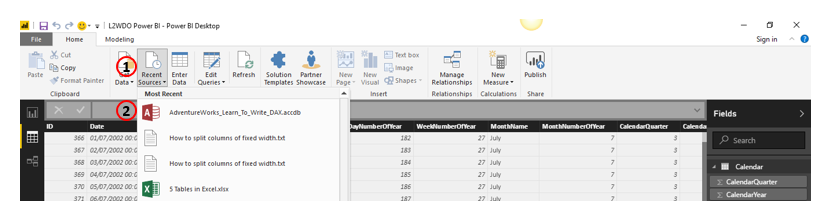3. In the Navigator (#3) dialog box that appears, select the dimProductSubCategory table (#4), and click on Load (#5).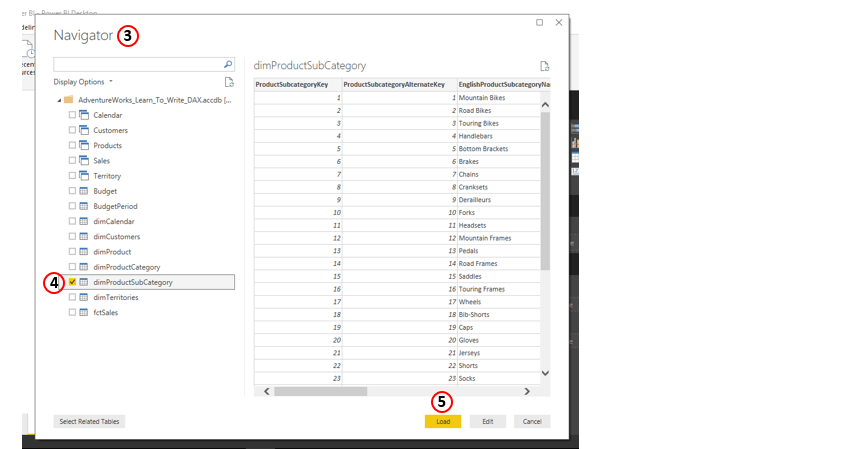The table will get loaded and will get connected to the data model.

4. Click on the Relationships view.  Rename the dimProductSubCategory table to SubCategory (#6) by double-clicking on the name of the table. (This is another way of renaming a table. You had already learnt how to rename a table in the Fields list). SubCategory is a lookup table of the Products table, so if you’re using the Collie layout methodology, you should place the SubCategory table above the Products table, as shown below (#7). You can observe that the existing Products table is connected to the new SubCategory table (#8).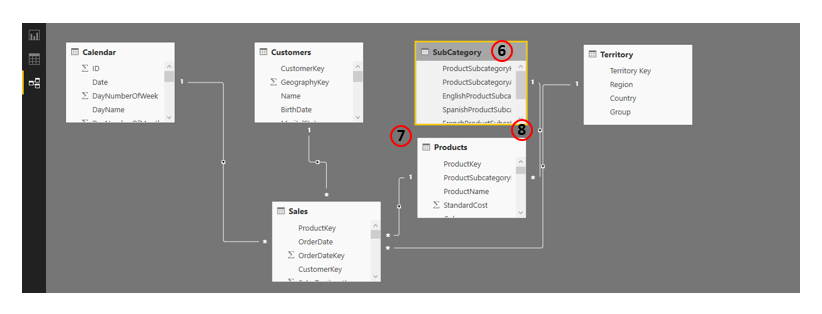# 3: Concept: Calculated Fields

In Power BI, DAX calculated fields are called measures. So, we refer to them as measures in this guide.

You can write a DAX measure in Power BI as follows:

• Right click on the table in the fields list (#1). g. Sales table.
• Select New measure.
• Write the measure in the formula bar (#2).
• Click the tick.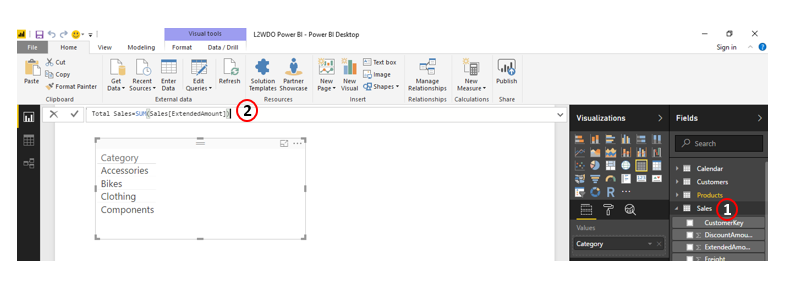• Immediately provide the formatting, so you don’t forget. You can find data formatting options (#2) under the Modelling tab (#1).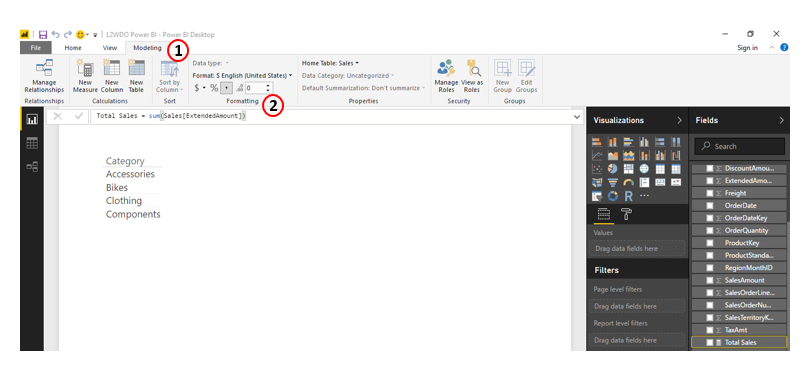To edit an existing measure, click on the three dots (…) to the right of the measure name in the fields list. The DAX formula for the measure appears in the formula bar, which you can edit.

# 4: DAX Topic: SUM(), COUNT(), COUNTROWS(), MIN(), MAX(), COUNTBLANK(), and DIVIDE()

## Changing the name of a measure

You can change the name of a measure as follows:

• Right-click on the measure in the Fields list.
• Select Rename.

The name turns into edit mode and you can type in the new name and press enter.

## Conditional Formatting

Steps to apply conditional formatting based on a Value:

• Select the Matrix.
• Right click on the Value (e.g. Count of Occupation) in the Values list.
• Click on Conditional Formatting (#1).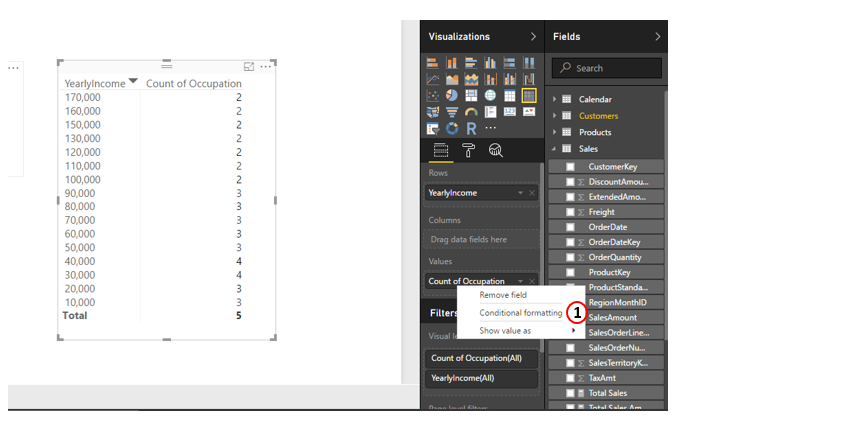## Here’s How: Moving an Existing Calculated Field to a Different Table

• Click on the measure in the Fields list (#1).
• The current table is displayed beside Home Table (#2).
• Click on the button to the right of the table name (#3).

List of the tables in the data model will be displayed.

• Click on the table where you want to move the measure.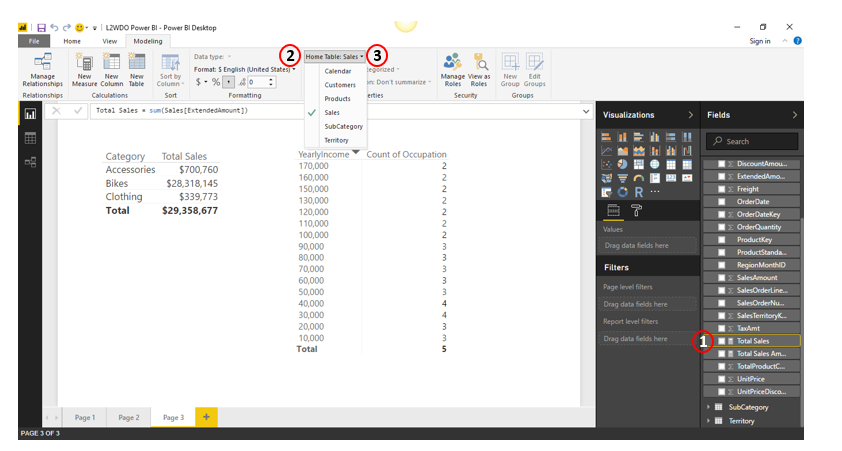# 7: DAX Topic: Calculated Columns

To create a calculated column, do the following:

• In the Fields list, click on the table in which you want to create the column. For e.g. Calendar (#1).
• Click on New Column (#2).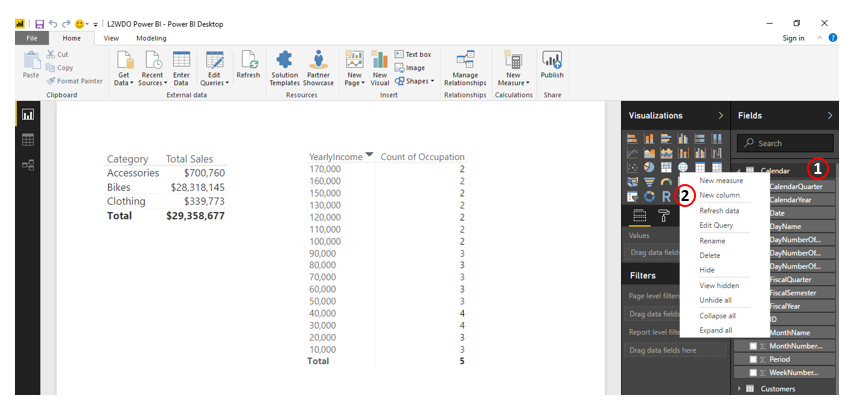• Write the following DAX formula in the formula bar (#3).

Is This a Weekend? = OR(Calendar[DayNumberOfWeek]=1,Calendar[DayNumberOfWeek]=7)

• Click the tick.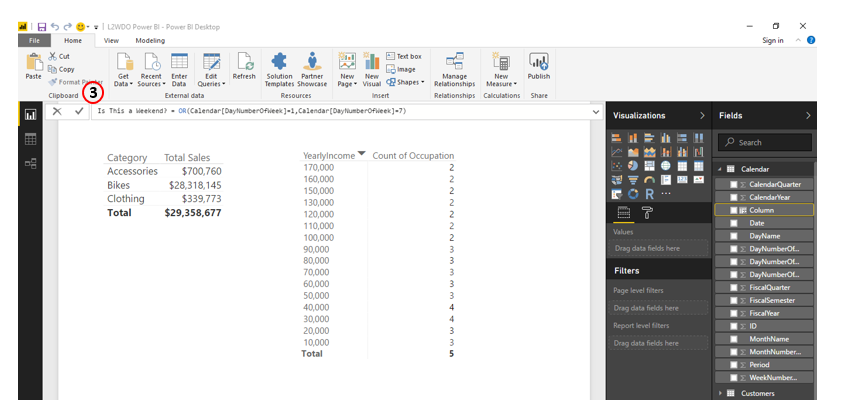# 17: Concept: KPIs and Multiple Data Tables

In Power BI Desktop, you can use the KPI visual for the visualisation of KPIs.

# 18: Concept: Cube Formulas

In Power BI Desktop, you still do not have the concept of Cube Formulas. However, keeping in view the utility of Cube Formulas, you can connect the Power BI Desktop to Excel, so that you can access the data model in Power BI Desktop from Excel.

Once you do this, you can create a Pivot Table in Excel based on your data model, and convert the Pivot Table to Cube Formulas as I explained in my book.

## Excel Workbook Connection to Local Power BI Desktop

Refer to my blog post on how to connect an Excel workbook to local Power BI Desktop here.

After the connection (#1 below), your Excel window looks as follows, wherein you have the Pivot Table (#2) that can be populated with the data in your Power BI Desktop data model, which is displayed in the Fields list (#3).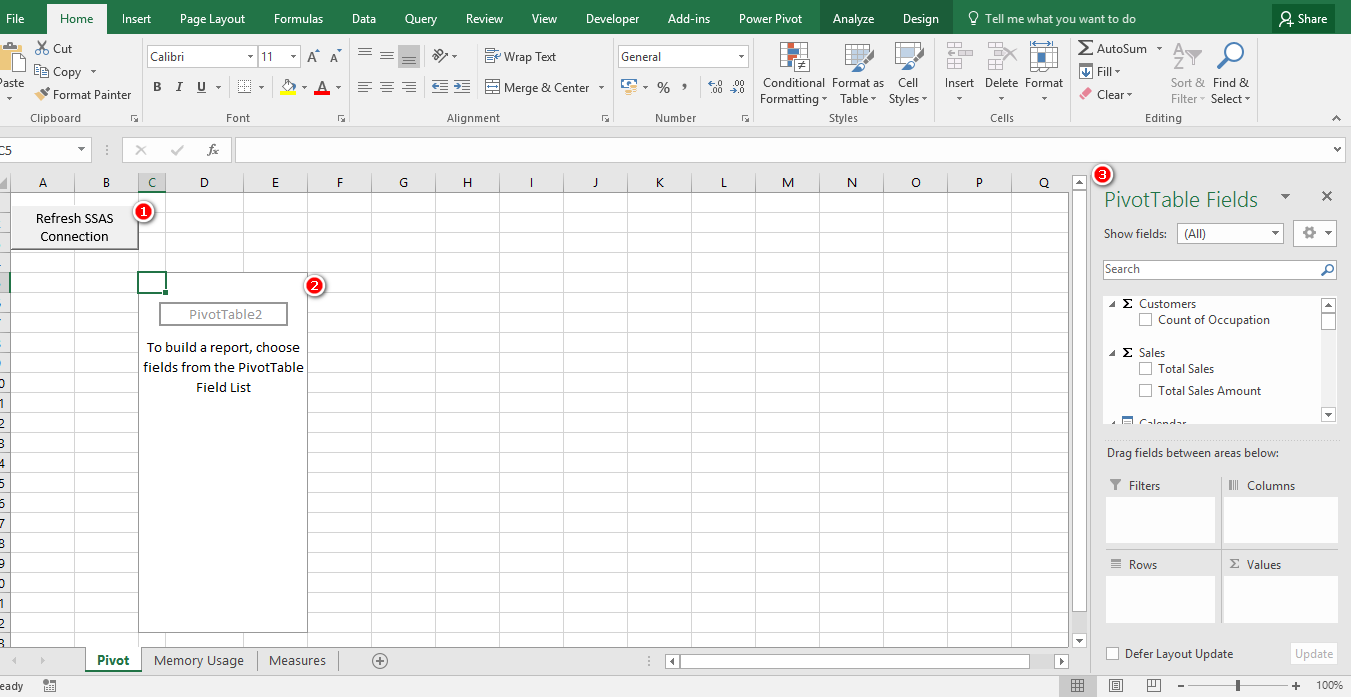Now, you can follow through the instructions in my book to understand cube formulas.

Scroll to Top

Power Query can automate boring, repetitive tasks you do every day.  Watch free previews of my online training course to see how.